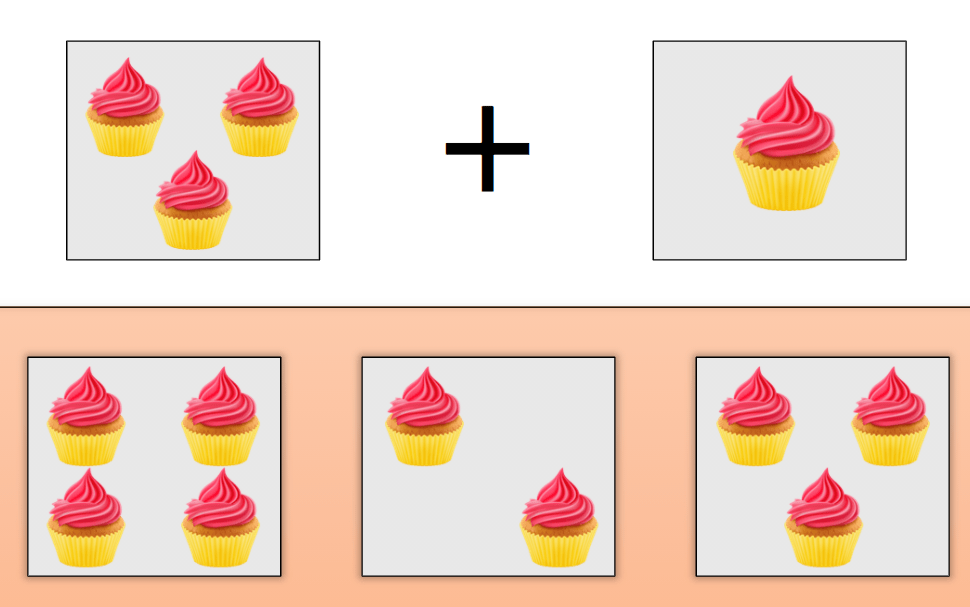# CBSE Class 1 Maths## Learn counting up to 20

In this course, students learn counting. Learning to count is not only about knowing the sequence of numbers, but also knowing the concept of counting. So, in this course, students learn to count by playing various educational games and interactive activities. The course has been broken down into the following sections: To teach the concept …## Learn Counting up to 10

In this course, students learn counting. Learning to count is not only about knowing the sequence of numbers, but also knowing the concept of counting. So, in this course, students learn to count by playing various educational games and interactive activities. The course has been broken down into the following sections: To teach the concept …## Subtraction upto 5

In this subtraction course, students will learn the concept of subtraction. The subtraction sums or problems will be limited upto subtraction from 5. These interactive subtraction worksheets or educational games will provide a simple method of learning about subtraction. With picture based subtraction, subtraction on a number line, some simple subtraction rules and some word …